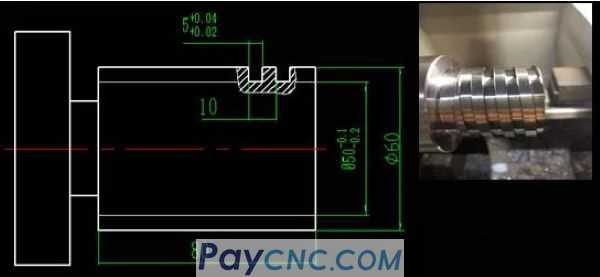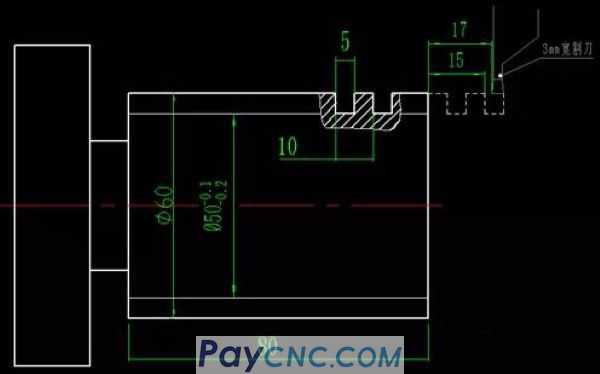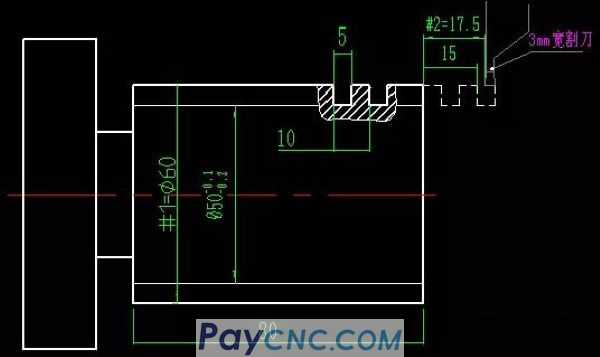Example of using "borrowing tool method" to write rectangular thread programSpecifically broken down into two parts: 1. "Layering" in the X direction 2. "Layering" in the Z direction   1. X-direction layering If the outer circle of the workpiece is D60 and the bottom diameter is D50, the program is relatively simple, so I won’t repeat it. Some programs are as follows: #1=60 N05#1=#1-0.4 IF[#1LE50]THEN#1=50 ... …(The threading section is omitted)… ... IF[#1 GT 50] GOTO05 (If you don’t have a car with a bottom diameter of 50, go back and continue the car)   Second, Z-direction stratificationIf the starting point of the thread cycle z of the 3mm cutter is set at a point outside the workpiece, part of the program is as follows: #2=17.5 N08#2=#2-0.5; IF[#2LE15]THEN#2=15 ... …(The threading section is omitted)… ... IF[#2 GT 15] GOTO08;   Is it simple? It is exactly the same as the above X-layered program, and thus completed the borrowing in the Z direction. At this point in the analysis, if you have a clearer mind, I will directly go to the program, as follows:% O16 (Use grooving tool, turning saw type thread program) N01 G54 S600 M03; (Set coordinate system, specify spindle speed, spindle forward rotation) N02 T0101; (tool location number and tool compensation number) N03 G00 X150 Z200; (rapidly reach the total starting point) N04 #1=60; (#1 represents the X command value of each layer of the car, assign #1=60 to represent the value of the 0 layer of the car) N05#2=[17+0.5] (#2 represents the Z command value of each tool of the lathe, and 17.5 represents the value of the 0 layer of the lathe) N06#1=#1-0.4; (Calculate the depth of the knife in X direction) N07 IF[#1LE 50]THEN#1=50 (to prevent overcutting in X direction) N08 G00X70 (knife lift) N09 #2=#2-0.5; (Calculate Z-direction tool borrowing value) N10IF[#2LE15]THEN#2=15 (to prevent over-cutting in Z direction) N11 G00 Z#2 (Z direction reaches the starting point of the thread cycle of the turning tool) N12 G00 X#1 (The X direction reaches the starting point of the thread cycle of the turning tool) N13 G32 Z-85 F10; (one cut) N14 IF[#2 GT 15]GOTO 08; (If there is no car to the left border, go back up and continue the car) N15 IF[#1 GT 50]GOTO 05 (If you don’t reach the bottom diameter of the thread, go back and continue the car) N16 G00 X150 N17 Z200 M05; N18 M30; %